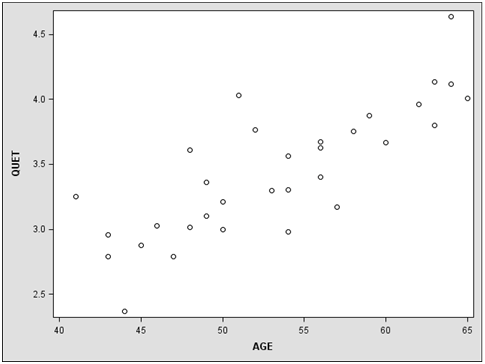# Biostatistics

1. The SAS dataset “112211” on blackboard gives the systolic blood pressure (SBP), body size (QUET), age (AGE), and smoking history (SMK = 0 if a nonsmoker, SMK = 1 if a current or previous smoker) for a hypothetical sample of 32 white males over 40 years old from the town of Angina. (QUET stands for “quetelet index,” a measure of size defined by QUET = 100 (weight/height2).)

a. On the accompanying scatter plot for QUET vs. AGE, sketch by eye a line that fits the data reasonably well. Comment on the relationship described.b. (1)

Determine the least-squares estimates of the slope ( 1) and intercept ( 0) for the

straight-line regression of QUET (Y) on AGE (X).

.          (2)  Sketch the estimated regression line on the scatter diagram in part (a) involving QUET and AGE. Compare this new line with the line you drew in part (a).

.          (3)  Test the null hypothesis of zero slope; be sure to interpret the result.

.          (4)  Based on your test in part (b)(3), would you conclude that body size increases

.          (5)  Obtain a 95% confidence interval for 1. In1terpret your result.

.          (6)  Would you reject the null hypothesis H0: = 0 in part (b)(3) based on the confidence

interval that you calculated in part (b)(5)? Explain.(7) Use SAS to find and draw 95% confidence bands and prediction bands with fitted

line on the scatter plot. (Must enable html output option in SAS: Tools->Options ->Preferences->Results->Check “Create HTML”.)

1

(8) Find the 99% confidence interval and prediction interval for an individual with AGE = 45 from SAS output. Interpret your answer.(9) Determine r and r2, and interpret your results, respectively.(10) Test H0: ρ = 0 versus H1: ρ ≠ 0 at α = .01, respectively. (Calculate by hand).

Field of study: